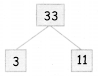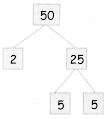Students can download 5th Maths Term 2 Chapter 2 Numbers Ex 2.2 Questions and Answers, Notes, Samacheer Kalvi 5th Maths Guide Pdf helps you to revise the complete Tamilnadu State Board New Syllabus, helps students complete homework assignments and to score high marks in board exams.

## Tamilnadu Samacheer Kalvi 5th Maths Solutions Term 2 Chapter 2 Numbers Ex 2.2

Question 1.

(i) The number divisible by 5 with no remainder
(a) 14
(b) 535
(c) 447
(d) 316
(b) 535(ii) Pick the number which is not a multiple of 6.
(a) 18
(b) 26
(c) 72
(d) 36
Hint:
(a) 18 = 6 × 3
(c) 72 = 8 × 9
(d) 36 = 6 × 6
(b) 26

(iii) The common multiple of 4 and 8 among the given number is.
(a) 32
(b) 84
(c) 68
(d) 76
Hint:
Multiples of 4 = 4, 8, 12, 16, 20, 24, 28, 32, 36, 40 ….
Multiples of 8 = 8, 16, 24, 32, 40, 48, 56, 64, …
Common multiples of 4, 8 is 8, 16, 24, 32, 40,…
(a) 32

(iv) Factors of 6
(a) 1, 2, 3
(b) 1, 6
(c) 1,2,3,6
(d) 2,3
Hint: Multiple of 9 are 9, 18, 27, 36, 45, 54, 63, 72, 81, 90, ……..
(c) 1, 2, 3, 6

(v) Multiple of 9 is
(a) 79
(b) 87
(c) 29
(d) 72
Hint: Multiple of 9 are 9, 18, 27, 36, 45, 54, 63, 72, 81, 90, …….
(d) 72Question 2.
Fill in the blanks:

Question 1.
Factors of 7 ______
1, 7

Question 2.
The only even prime number is ______
1,2

Question 3.
L.C.M of 4, 12 ______
12

Question 4.
L.C.M of 5, 15 ______
15

Question 5.
The numbers which divides 35 without reminders are _____, ______, ______.
1, 5, 7Question 3.
Write down the factors of the given numbers.
(i) 25
(ii) 36
(iii) 14
(iv) 16
(v) 12
(i) 25
Factors of 25 is 1, 5, 25

(ii) 36
Factors of 36 is 1, 2, 3, 4. 6, 9, 12, 18, 36

(iii) 14
Factors of 14 is 1, 2, 7, 14

(iv) 16
Factors of 16 is 1, 2, 4, 8, 16

(v) 12
Factors of 1, 2, 3. 4, 6, 12Question 4.
Draw a picture of factor tree.

(i) 18
(ii) 33
(iii) 16
(iv) 50
(i) 18(ii) 33(iii) 16(iv) 50Question 5.
Write down the first 5 multiples of the given numbers.
(i) 7
(ii) 9
(iii) 16
(iv) 11
(v) 21
(i) 7
= 7 Multiple of 7 – 7, 14, 21, 28, 35

(ii) 9
= 9 Multiple of 9 – 9, 18, 27, 36, 45

(iii) 16
= 16 Multiple of 16 – 16, 32, 48, 64, 80

(iv) 11
= 11 Multiple of 11 is 11, 22, 33, 44, 55

(V) 21
= 21 Multiple of 21 is 21, 42, 63, 84, 105Question 6.
Find first three common multiples of the given numbers.
(i) 24, 16
(ii) 12,9
(iii) 24,36
(i) 24, 16
Multiples of 24 = 24, 48, 72, 96, 120, 144, 168, 192, 216, 240, 264
Multiples of 16 = 16, 32, 48, 64, 80, 96, 112, 128, 144, 160, 176, 192. 208, 224, 240, 256
Common multiples of 24 and 16 is 48, 96, 144, 192, 240

(ii) 12, 9
Multiples of 12 = 12, 24, 36, 48, 60, 72, 84, 96, 108, 120,
Multiples of 9 – 9, 18, 27, 36, 45, 54, 63, 72, 81, 90, 99, 108, 117
Common multiples of 12 and 9 is 36, 72, 108

(iii) 24, 36
Multiples of 24 = 24, 48, 72, 96, 120, 144, 168, 192, 216,
Multiples of 36 = 36, 72, 108, 144, 180, 216
Common multiples of 24 and 36 is 72, 144, 216Question 7.
Find L. C.M of the given numbers.
(i) 12 and 28
(ii) 16 and 24
(iii) 8 and 14
(i) 12 and 28
Multiples of 12 = 12, 24, 36, 48, 60, 72, 84, 96, 108, 120, 132, 144, 156, 168, 180
Multiples of 28 = 28, 56, 84, 112, 140, 168, 196, ……
Common multiples of 12 and 28 is 84, 168, ……..
LCM is 84

(ii) 16 and 24
Multiples of 16 = 16, 32, 48, 64, 80, 96, 112, 128, 114, …….
Multiples of 24 = 24, 48, 72, 96, 120, 144, ……
Common multiples of 16 and 24 is 48, 96, 144, ……..
LCM is 48

(iii) 8 and 14
Multiples of 8 = 8, 16, 24, 32, 4O48, 56, 64, 72, 80, 88, 96, 104, 112, …
Multiples of 14 = 14, 28, 42, 56, 70,84 98 112 ……
Common multiples of 8 and 14 is 56, 112, …….

(iv) 30 and 20
Multiples of 30 = 30, 60, 90, 120, 150, 180, 210, 240, 270, …
Multiples of 20 = 20, 40, 60, 80, 100, 120, 140, 160, 180, …
Common multiples of 30 and 20 is 60, 120, 180
LCM of 30 and 20 is 60.The least common multiple (LCM) of 12 and 18 is 36.

Question 8.
Ramya visits the gym five days once and Kavitha visits the gym six days once. In which day will they meet each other?